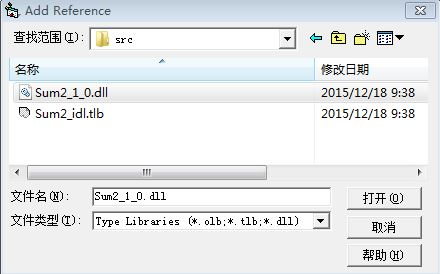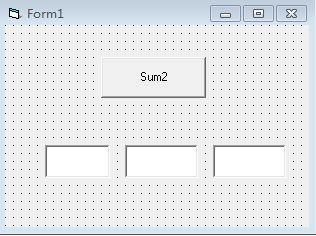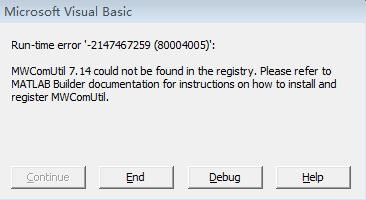MATLAB 里 的 M文件的内容是
function c=Sum2(a,b)
c=a+b;
endVB代码里面是：
Private a As Double
Private b As Double
Private c As Double
Private MySum2 As Sum2.Sum2

Private Sub CommandSum2_Click()
Text3.Text = MySum2(Text1.Text, Text2.Text)
End Sub

Set MySum2 = New Sum2.Sum2
End Sub1个回答